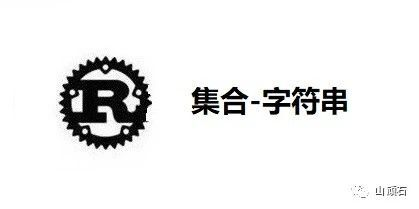# Rust 从 0 到 1- 集合 - 字符串• Rust 设计上倾向于提前发现可能的错误

• String 的数据结构比它表面上看上去复杂的多

• UTF-8 字符集

#### 什么是字符串

String 类型是由标准库提供的，并没有被写入 Rust 语言的核心部分，它是可增长的、可变的、有所有权的、UTF-8 编码的字符串类型。当我们谈到 Rust 的 “字符串”时，通常指的是 String 和字符串切片引用 &str 两者。虽然本章节我们讨论的内容主要是关于 String 的，不过这两种类型在 Rust 标准库中都经常被用到，并且他们都是基于 UTF-8 编码的。

Rust 标准库中还包含有其他字符串类型，比如 OsString、OsStr、CString 和 CStr 等。Crate 库上还有更多字符串类型的变体选择，它们名字的结尾 String 或是 Str 对应着它们是具有所有权还是借用，就像 String 和 str，这些字符串类型的变体能够以不同的编码，或者内存使用上以不同的形式等来存储字符串文本内容。他们的使用方式和适合场景请参考对应的 API 文档，我们在此不会讨论。

#### 创建一个字符串

let mut s = String::new();

let data = "initial contents";let s = data.to_string();// the method also works on a literal directly:let s = "initial contents".to_string();

let s = String::from("initial contents");

let hello = String::from("السلام عليكم");let hello = String::from("Dobrý den");let hello = String::from("Hello");let hello = String::from("שָׁלוֹם");let hello = String::from("नमस्ते");let hello = String::from("こんにちは");let hello = String::from("안녕하세요");let hello = String::from("你好");let hello = String::from("Olá");let hello = String::from("Здравствуйте");let hello = String::from("Hola");

#### 修改字符串

##### 使用 push_str 和 push 拼接字符串

let mut s = String::from("foo");s.push_str("bar");

let mut s1 = String::from("foo");let s2 = "bar";s1.push_str(s2);println!("s2 is {}", s2);

push 方法的参数为一个单独的字符，并将其拼接到 String 中：

let mut s = String::from("lo");s.push('l');

##### 使用 + 或 format! 拼接字符串

let s1 = String::from("Hello, ");let s2 = String::from("world!");let s3 = s1 + &s2; // 注意 s1 被移动了，不能继续使用

fn add(self, s: &str) -> String {

let s1 = String::from("tic");let s2 = String::from("tac");let s3 = String::from("toe");let s = s1 + "-" + &s2 + "-" + &s3;

let s1 = String::from("tic");let s2 = String::from("tac");let s3 = String::from("toe");let s = format!("{}-{}-{}", s1, s2, s3);

#### 通过索引访问字符串

let s1 = String::from("hello");let h = s1;

##### 内部实现

String 实际上是基于 Vec<u8> 进行的封装。我们先来看一个例子：

let len = String::from("Hola").len();

len 的值是 4 ，这意味着储存字符串 Hola 的 Vec 的长度是四个字节，即每个字母的 UTF-8 编码都占用一个字节。接着来看下面这个例子（注意这个字符串中的首字母是西里尔字母的 Ze 而不是阿拉伯数字 3 ）：

let len = String::from("Здравствуйте").len();

let hello = "Здравствуйте";let answer = &hello;

answer 的值应该是什么？是第一个字符 З 吗？当使用 UTF-8 编码时，З 的第一个字节是 208，第二个字节是 151，因此 answer 对应的应该是 208，不过 208 并不是一个有效的字母。因此，通常返回 208 并不是我们预期的结果，但它确实是 Rust 在索引 0 的位置所存储的数据。我们通常不会想要返回一个字节中存储的值。为了避免这种意外以及造成潜在的缺陷，Rust 在编译时就不允许这些代码通过。

##### 字节、标量值和字形簇

[224, 164, 168, 224, 164, 174, 224, 164, 184, 224, 165, 141, 224, 164, 164,224, 165, 135]

['न', 'म', 'स', '्', 'त', 'े']

["न", "म", "स्", "ते"]

Rust 提供了多种不同的方式来展现计算机储存的原始字符串数据，这样我们就可以根据需要选择不同的表现方式，而不管是使用的那种人类语言。

#### 将字符串切片

let hello = "Здравствуйте";let s = &hello[0..4];

#### 遍历字符串

for c in "नमस्ते".chars() {    println!("{}", c);}

bytes 方法返回每一个原始字节：

for b in "नमस्ते".bytes() {    println!("{}", b);}

#### 字符串并不简单### 山

IT老兵，关注Rust、Java、前端、架构、大数据、AI、算法等；平时喜欢美食、旅游、宠物、健身、篮球、乒乓球等。希望能和大家交流分享。

## 评论